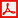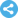Computational and Applied Mathematics Journal
Manuscript Information

Numerical Solution of Boundary Value Problems for the Laplacian in R3 in the Case of Complex Boundary Surface
Computational and Applied Mathematics Journal
Vol.1 , No. 2, Publication Date: Feb. 15, 2015, Page: 29-35
1183 Views Since February 15, 2015, 448 Downloads Since Apr. 12, 2015Paper in PDF (301K)Follow on

Authors

  Olexandr Polishchuk, Department of Nonlinear Mathematical Analysis, Pidstryhach Institute for Applied Problems of Mechanics and Mathematics, National Academy of Sciences of Ukraine, Lviv, Ukraine.

Abstract

Potential theory is one of the ways to solve the boundary value problems for the Laplacian. Well-posed solvability of integral equations equivalent to boundary value problems allow to use for their solution well known projection methods. In many applied problems the boundary surfaces have complex geometry and contain the edges and corner points. Together with the singularity in the kernel this gives rise to a singularity in the searched density of the potential. The methods are proposed for removal of singularities in the kernels and unknown densities of potentials that significantly improve the accuracy of projection methods, as well as their numerical solution.

Keywords

Boundary Integral Equations, Projection Methods, Collocation, Removal of Singularity

Reference

  T. V. Hromadka II and C. Lay, The complex variable boundary elements method in engineering analysis, Springer-Verlag, New York, USA, 1987.  G. C. Hsiao and W. L. Wendland, Boundary integral equations, Springer, Berlin, Germany, 2008.  J. C. Nedelec and J. Planchard, “Une methode variationnelle d’elements finis pour la resolution numerique d’un probleme exterieur dans R3”, R.A.I.R.O., R3, no.7, pp.105-129, 1973.  J. Giroure, “Formulation variationnelle par equations integrales de problemes aux limites exterieurs”, Rapport Interne du Centre de mathematiques Appliquees de l’Ecole Politechnique, no. 6, 1976.  A.D. Polishchuk, “Solution of the double-sided Dirichlet-Neumann problem for the Laplacian in R3 by means of the potential theory methods “, Mathematical Methods and Physicomechanical Fields, vol. 48, no. 1, pp. 59-64, 2004.  A. D. Polishchuk, “Construction of boundary operators for the Laplacian”, Proceedings of the 17th International Seminar “Direct and Inverse Problems of Electromagnetic and Acoustic Wave Theory”, Lviv, Ukraine, September 12-15, 2005, pp. 137-142.  A. D. Polishchuk, “Construction of boundary operators for the Laplacian in the case of tired boundary surface”, Proceedings of the 11th International Seminar “Direct and Inverse Problems of Electromagnetic and Acoustic Wave Theory”, Tbilisi, Georgia, 12-13 October 2006, pp.153-160.  A. D. Polishchuk, “Solution of double-sided boundary value problems for the Laplacian in R3 by means of potential theory methods”, Proceedings of the 17th International Seminar “Direct and Inverse Problems of Electromagnetic and Acoustic Wave Theory” Tbilisi, Georgia, September 22-25, 2014, pp. 140-142.  P. Kythe and P. Puri, Computational Methods for Linear Integral Equations, Springer Science & Business Media, 2002.  A. G. Ramm, “A collocation method for solving integral equations”, International Journal of Computing Science and Mathematics, vol. 2, no. 3, pp. 222-228, 2009.  J. Rashidinia and M. Zarebnia, “Convergence of approximate solution of system of Fredholm integral equations”, Journal of Mathematical Analysis and Applications, vol. 333, no. 2, pp. 1216–1227, 2007.  K. Maleknejad, N. Aghazadeh, R. Mollapourasl, “Numerical solution of Fredholm integral equation of the first kind with collocation method and estimation of error bound”, Applied Mathematics and Computation, vol. 179, no. 1, pp. 352–359, 2006.  A. D. Polishchuk, “About numerical solution of some integral equations of the first kind”, Proceedings of the 17th International Seminar “Direct and Inverse Problems of Electromagnetic and Acoustic Wave Theory”, Tbilisi, Georgia, 24-27 September 2012, pp.159-166.  A. I. Grebennikov, “Methods of spline-collocation and double spline-approximation for solution of operator equations and its application to solution of integral equations with singularities”, In: Methods and algorithms in numerical analysis, Nauka, Moscow, USSR, pp.128-140, 1984.  A. G. Kyurkchan and N. I. Smirnova, “Solution of wave diffraction problems by the method of continued boundary conditions”, Acoustic Journal, vol. 53, no. 4, pp. 490-499, 2007.  G. Fiсhera, “Asymptotic behavior of the electric field and density of the electric charge in the neighborhood of singular points of a conducting surface”, Russian Mathematical Surveys, vol. 30, no. 3, pp.105-124, 1975.  A. V. Amenitskiy, “Boundary integral equations for nanlizing dynamic problems of 3-D porouseelasticity”, Problems of Strenght and Plasticity, vol.30, pp.164-171, 2009.  A. V. Ivanov and M. A. Tiunov, “Use of boundary integral equations method during modeling of formation and dynamic of sheaf in installation of the electronic cooling”, Bulletin of Novosibirsk State University, Physics, vol. 3, no. 1, pp. 56-61, 2008.  M. Y. Medvedyk, “Collocation method for solution of the problem of diffraction of electromagnetic waves by a dielectric body, located on the resonator”, Bulletin of High School, Volga Region, Physics and Mathematics, vol. 2, pp.28-40, 2011.  H. Triebel, Theory of function spaces, Academische Verlagsgesellschaft Geest @ Postig K.-G., Leibzig, Germany, 1983.  V. I. Krylov, Approximate calculation of integrals, The Macmillan Co, New York, USA, 1962.  I. V. Boikov, “Asymptotically optimal algorithms for calculation of singular integrals”, In: Use of numerical methods in scientific investigations, Penza state University Press, Penza, USSR, pp.3-10, 1983.  H. V. Stoll and P. Strauss, “On the numerical integration of certain singular integrals”, Computing, vol. 48, pp. 177-189, 1992.